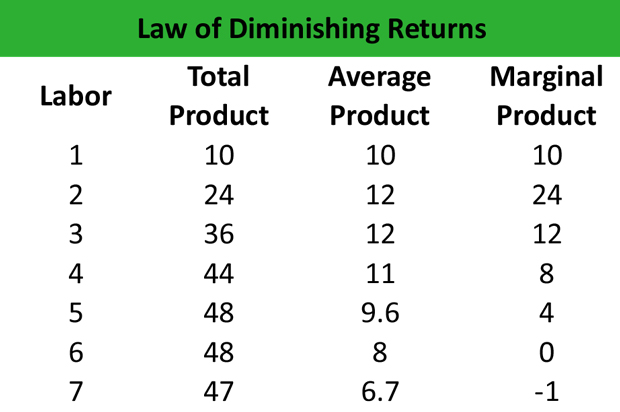# What is the Law of Diminishing Returns?

Definition: The law of diminishing returns is an economic concept that shows that there is a point where an increased level of inputs does not equal to an equal increase level of outputs. In other words, after a certain point of production each input will not increase outputs at the same rate. There will be a diminishing effect where each input contributes less in proportion to the overall production output.

## What Does the Law of Diminishing Returns Mean?

What is the definition of the law of diminishing returns? The law of diminishing returns is explained by the fact that as the variable factor increases; a lower proportion of the fixed factor corresponds to each unit. In the beginning, when the variable factor is at relatively low levels, the average and marginal return is equally low because the fixed factors may not be fully utilized, thereby eliminating an opportunity for specialization.

However, as the variable factor keeps increasing and new units are added, the average and marginal returns start diminishing after a certain point because the increase in the quantity of the variable factor diminishes the marginal return of the fixed factors. This concept can also be applied to consumption.

Let’s look at an example.

## Example

The following table shows the total product, the average product and the marginal product of a firm operating in the short-term. The variable factor is the labor.Observations

1. When the marginal product increases, the total product grows by the same amount. (L=1, MP =10, TP=10, L=2, MP=24, TP=24).

2. At the point where the marginal product reaches its maximum value (L=2, MP=24), the total product starts to increase at a decreasing rate. That is, the law of diminishing returns comes into effect after adding an additional unit of labor (L=3).

3. When the marginal product is equal to 0, the total product takes the maximum value (TP=48).

4. When the marginal product is negative, the total product decreases (TP=47).

5. When the marginal product is equal to or higher than the average product, the average product increases as the variable factor increases (L=1, L=2).

6. When the marginal product is equal to or lower than the average product, the average product decreases as the variable factor increases (L=3 to L=7).

## Summary Definition

Define The Law of Diminishing Returns: The law of diminishing returns states that each additional input will less and less output as more are added.